# Intervention Image 圖片處理

## 原始圖片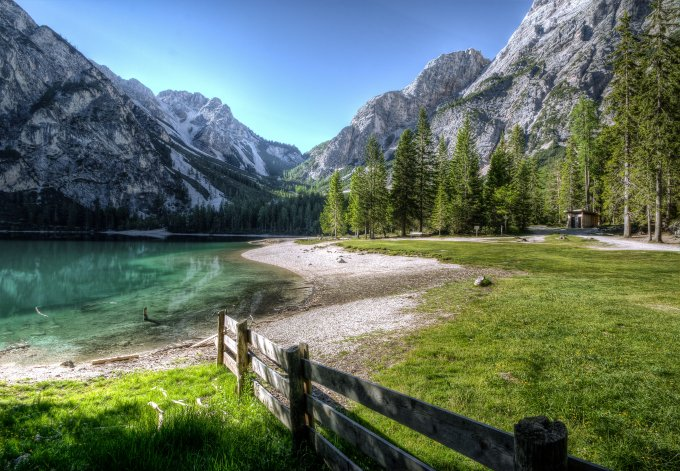## resize() 圖片縮放

``````// 建立圖片實例
\$img = Image::make('public/foo.jpg');

// 重新調整圖片寬高至 300x200
\$img->resize(300, 100);
``````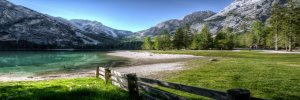resize to 300x100

``````// 寬度調整至 300px，高度不變
\$img->resize(300, null);
``````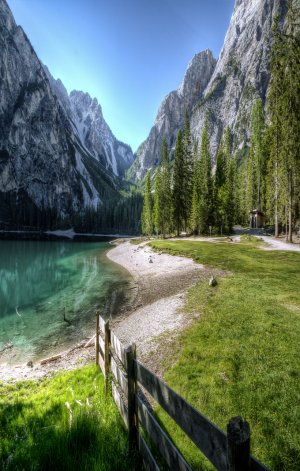resize to 300xnull

``````// 高度調整至 200px，寬度不變
\$img->resize(null, 100);
``````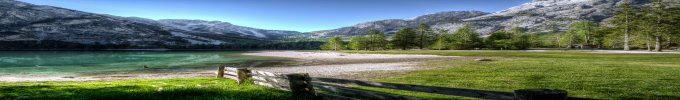resize to nullx100

``````// 固定寬 300px，高度等比例縮放
\$img->resize(300, null, function (\$constraint) {
\$constraint->aspectRatio();
});
``````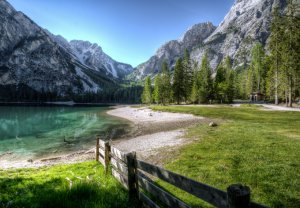resize to 300xnull aspectRatio

``````// 固定高 100px，寬度等比例縮放 width)
\$img->resize(null, 100, function (\$constraint) {
// 等比例縮放
\$constraint->aspectRatio();
});
``````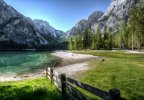resize to nullx100 aspectRatio

``````// 固定寬 300px, 高 100px，寬度等比例縮放
\$img->resize(300, 100, function (\$constraint) {
// 等比例縮放：若兩個寬高比例與原圖不符的話，會以最短邊去做等比例縮放
\$constraint->aspectRatio();
});
``````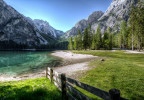resize to 300x100 aspectRatio

``````\$img->resize(null, 1000, function (\$constraint) {
// 等比例縮放
\$constraint->aspectRatio();
// 若圖片較小，不需要將圖片放大
\$constraint->upsize();
});
``````resize to nullx1000 aspectRatio & upsize

## crop() 圖片裁切

``````// 建立圖片實例
\$img = Image::make('public/foo.jpg');

// 裁切圖片寬度 100px，高度 300px，從圖片中間為中心點開始裁切
\$img->crop(100, 300);
``````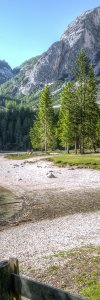\$img->crop(100, 300);

``````// 從圖片左上角為原點 0,0，然後裁切圖片寬度 100px，高度 300px
\$img->crop(100, 300, 0, 0);
``````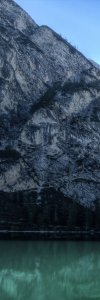\$img->crop(100, 300);

``````// 從圖片左上角為原點，往右位移 35px，往下位移 75px，然後裁切圖片寬度 100px，高度 300px
\$img->crop(100, 300, 35, 75);
``````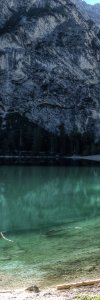\$img->crop(100, 300, 35, 75);

## blur() 模糊

``````// 建立圖片實例
\$img = Image::make('public/foo.jpg');

// 輕微的模糊圖片（預設強度 1）
\$img->blur();
``````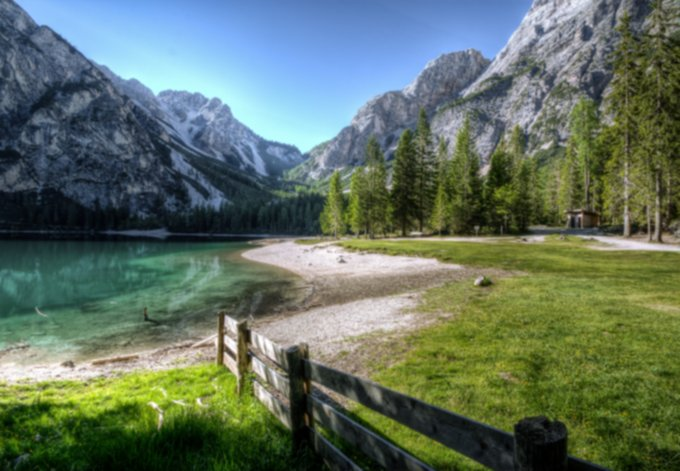\$img->blur();

``````// 指定模糊的強度
\$img->blur(15);
``````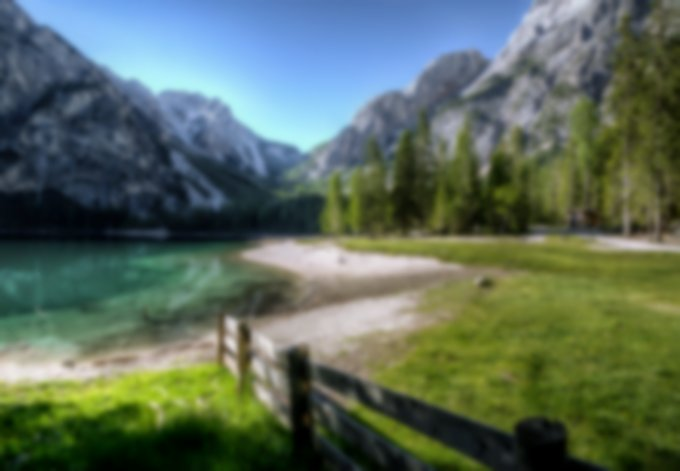\$img->blur(15);

## brightness() 亮度

``````// 建立圖片實例
\$img = Image::make('public/foo.jpg');

// 增加圖片亮度 35
\$img->brightness(35);
``````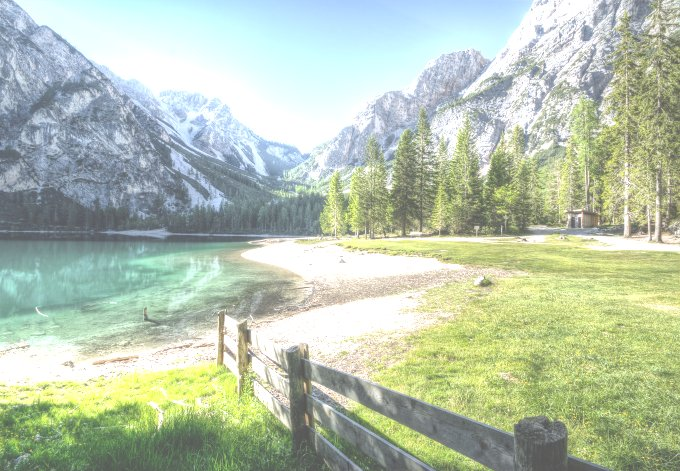\$img->brightness(35);

``````// 減低圖片亮度 -5，再減低亮度 -25
\$img->brightness(-5)->brightness(-25);
``````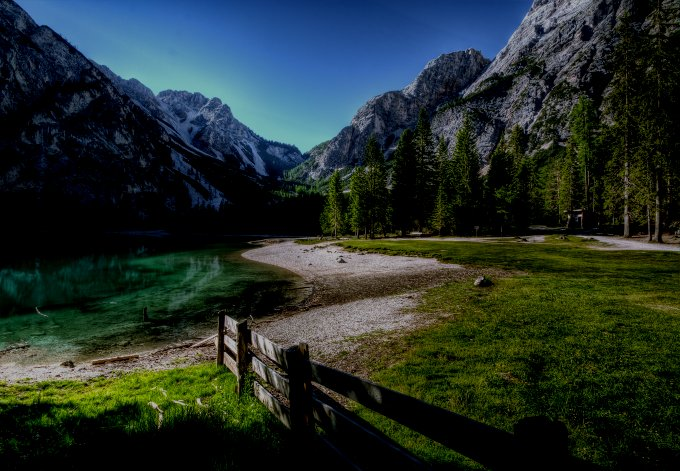\$img->brightness(-5)->brightness(-25);

## colorize() 顏色增減

``````// 建立圖片實例
\$img = Image::make('public/foo.jpg');

// 紅色 -100
\$img->colorize(-100, 0, 0);
``````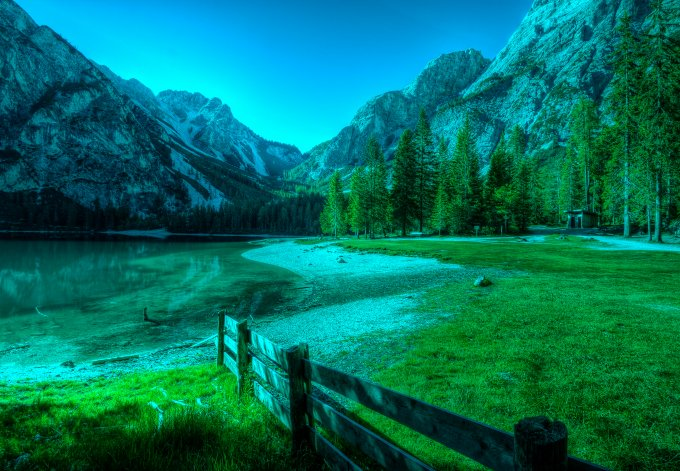\$img->colorize(-100, 0, 0);

``````// 紅色 100
\$img->colorize(100, 0, 0);
``````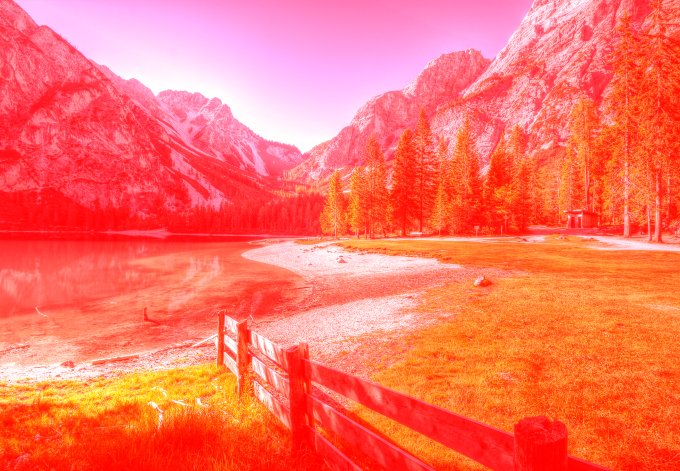\$img->colorize(100, 0, 0);

``````// 綠色 30
\$img->colorize(0, 30, 0);
``````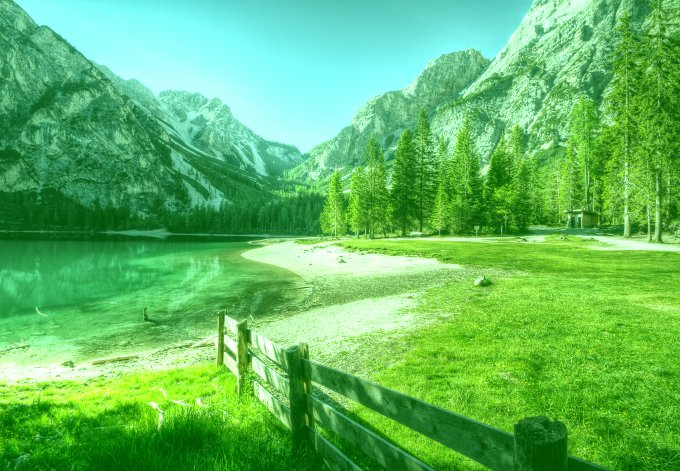\$img->colorize(0, 30, 0);

## contrast() 對比

``````// 建立圖片實例
\$img = Image::make('public/foo.jpg');

// 增加對比 65
\$img->contrast(65);
``````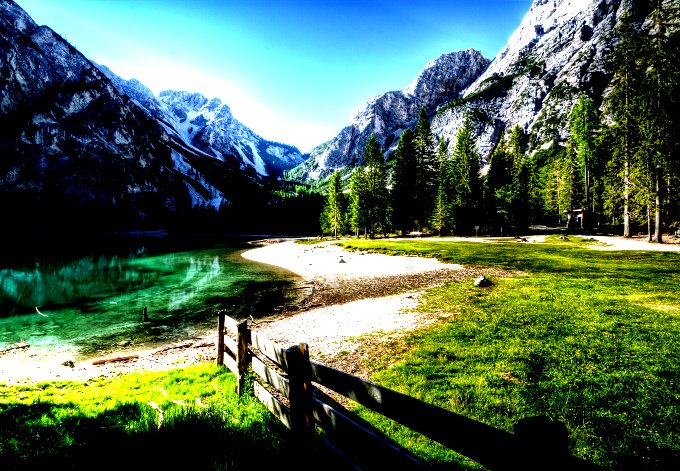\$img->contrast(65);

``````// 增加對比 100
\$img->contrast(100);
``````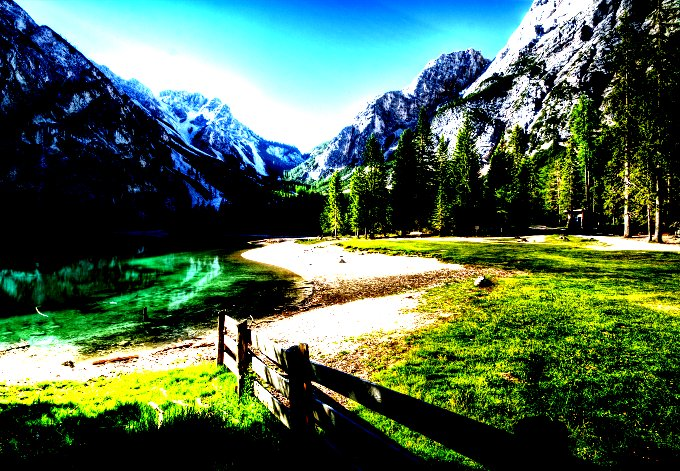\$img->contrast(100);

``````// 減少對比 35
\$img->contrast(-35);
``````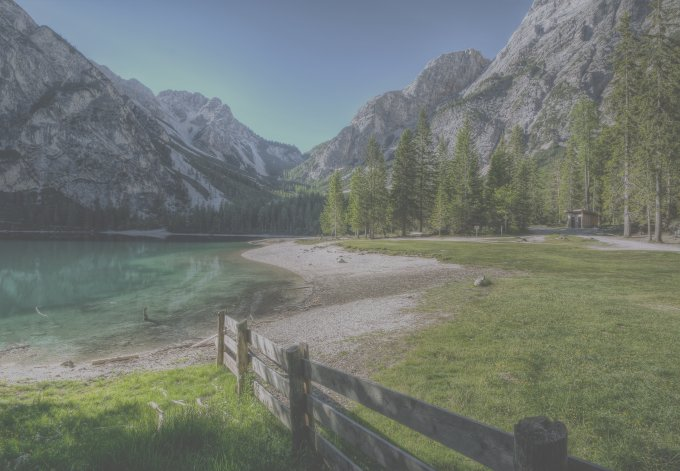\$img->contrast(-35);

## encode() 編碼

• jpg — return JPEG encoded image data
• png — return Portable Network Graphics (PNG) encoded image data
• gif — return Graphics Interchange Format (GIF) encoded image data
• tif — return Tagged Image File Format (TIFF) encoded image data
• bmp — return Bitmap (BMP) encoded image data
• ico — return ICO encoded image data
• psd — return Photoshop Document (PSD) encoded image data
• webp — return WebP encoded image data
• data-url — encode current image data in data URI scheme (RFC 2397)
``````// 將 png 圖片轉為 jpg，並使用 quality 75 的圖片
\$jpg = (string) Image::make('public/foo.png')->encode('jpg', 75);

// 將 png 圖片轉為網址 base64 的圖片
\$data = (string) Image::make('public/bar.png')->encode('data-url');
``````

## flip() 翻轉

``````// 建立圖片實例
\$img = Image::make('public/foo.jpg');

// 垂直翻轉
\$img->flip('v');
``````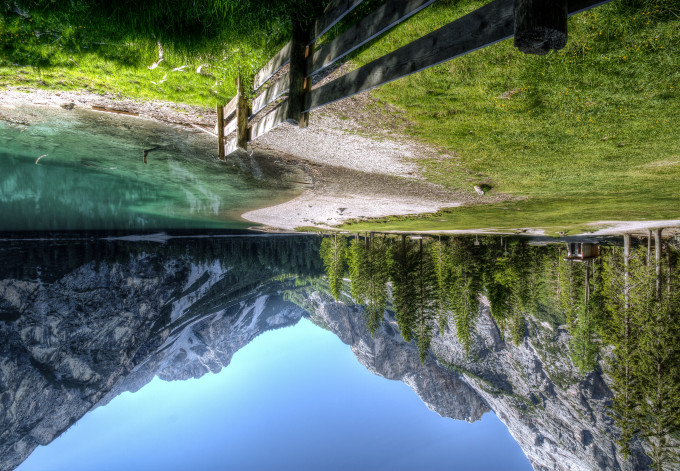\$img->flip(‘v’);

``````// 水平翻轉
\$img->flip('h');
``````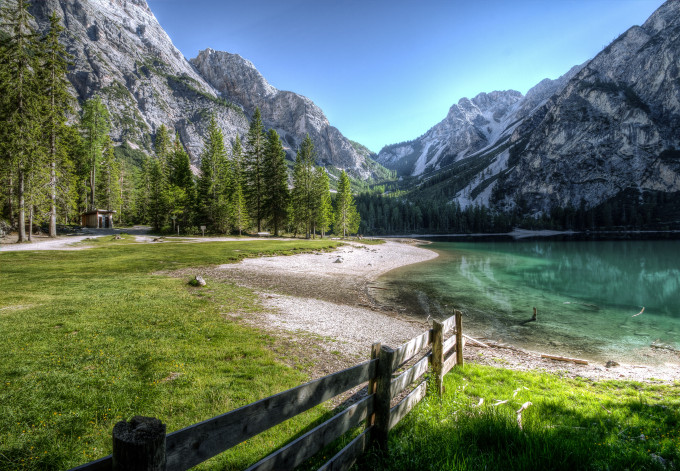\$img->flip(‘h’);

``````// 水平+垂直翻轉
\$img->flip('h')->flip('v');
``````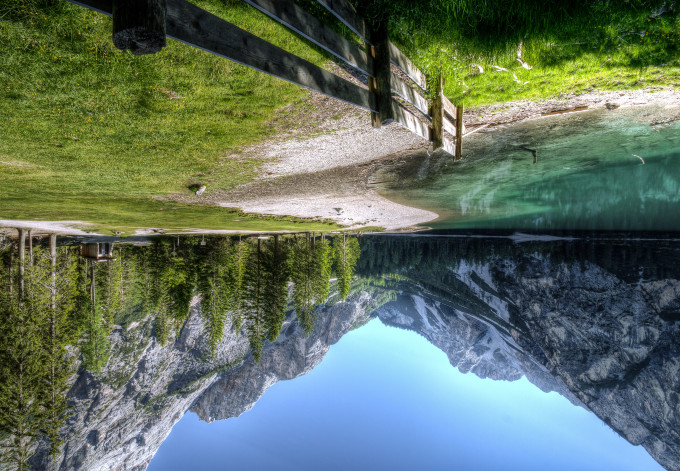\$img->flip(‘h’)->flip(‘v’);

## fit() 裁切(crop) + 圖片縮放(resize)

``````// 建立圖片實例
\$img = Image::make('public/foo.jpg');

// 圖片固定圖片比例，縮放至 300x100，並將將超過 300x100 的部分裁切掉
\$img->fit(300,100);
``````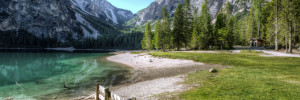\$img->fit(300,100);

``````// 圖片使用 300x300 的 1:1 比例，將圖片填滿
\$img->fit(300);
\$img->fit(300,300);
``````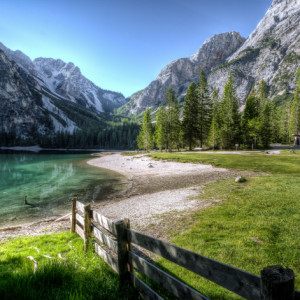\$img->fit(300);

``````// 圖片固定圖片比例，縮放至 1200x1000，並將將超過 1200x1000 的部分裁切掉，且避免圖片放大超過原本圖片尺寸
\$img->fit(1200, 1000, function (\$constraint) {
// 避免圖片放大超過原本尺寸
\$constraint->upsize();
});
``````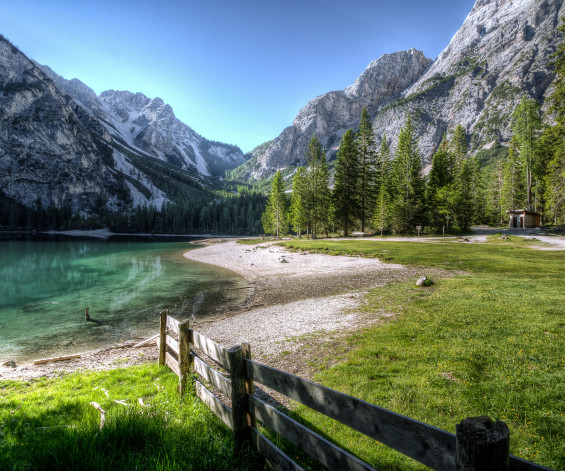\$img->fit(1200, 1000); upsize()

## gamma() Gamma 校正

``````// 建立圖片實例
\$img = Image::make('public/foo.jpg');

// Gamma 校正 1.6
\$img->gamma(1.6);
``````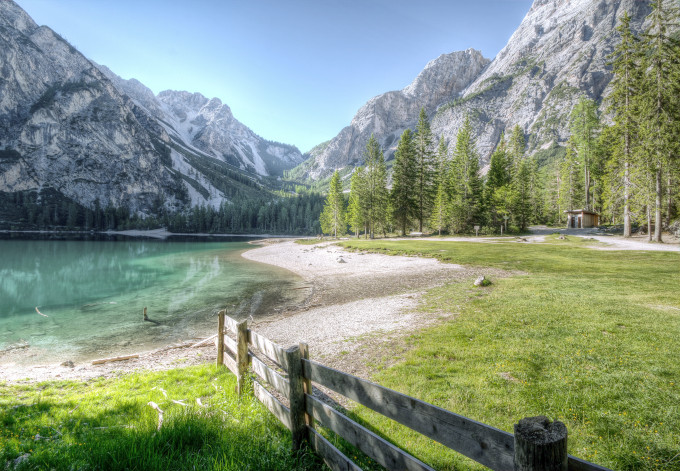\$img->gamma(1.6);

``````// Gamma 校正 1/2.2
\$img->gamma(1 / 2.2);
``````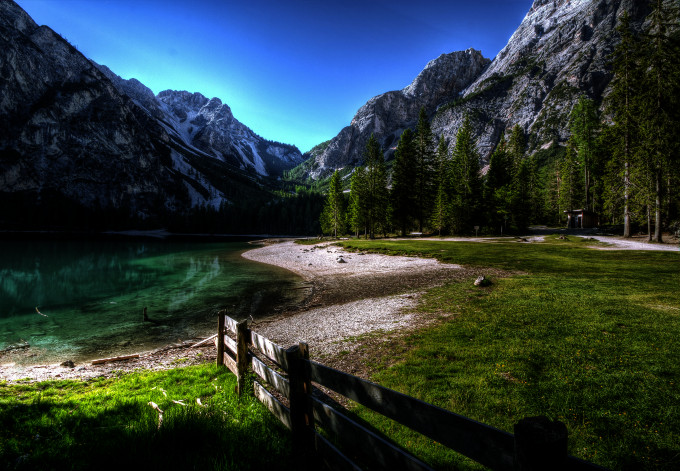\$img->gamma(1 / 2.2);

## greyscale() 灰階

``````// 建立圖片實例
\$img = Image::make('public/foo.jpg');

// 灰階
\$img->greyscale();
``````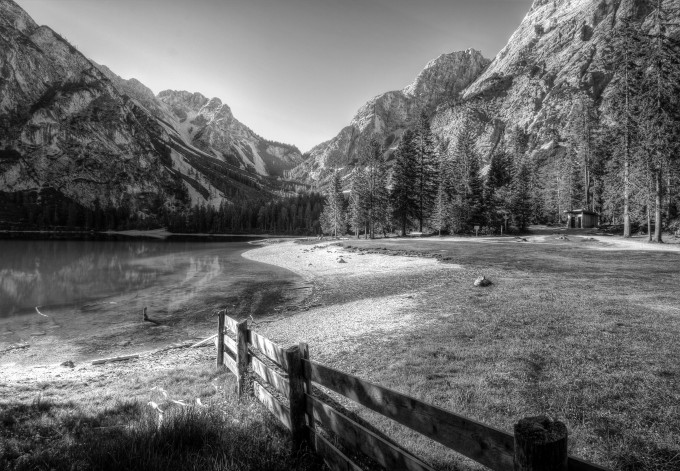\$img->greyscale();

## heighten() 重新縮放圖片，以圖片高度為主，等比例縮放

``````// 圖片高度縮放至 100px，並依照原本圖片比例縮放
\$img = Image::make('public/foo.jpg')->heighten(100);
``````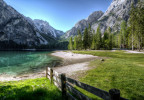\$img->heighten(100);

``````// 圖片高度縮放至 1000px，並依照原本圖片比例縮放，不將原本圖片放大
\$img = Image::make('public/foo.jpg')->heighten(1000, function (\$constraint) {
// 若圖片較小，不需要將圖片放大
\$constraint->upsize();
});
``````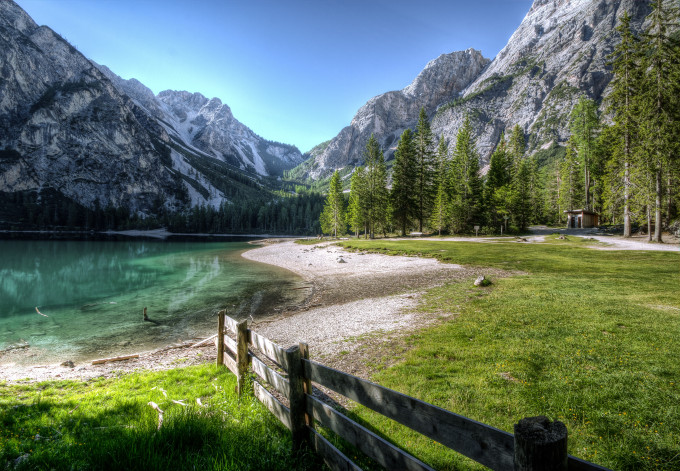\$img->heighten(1000); upsize()

## widen() 重新縮放圖片，以圖片寬度為主，等比例縮放

``````// 圖片寬度縮放至 100px，並依照原本圖片比例縮放
\$img = Image::make('public/foo.jpg')->widen(100);
``````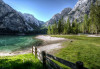\$img->widen(100);

``````// 圖片寬度縮放至 1000px，並依照原本圖片比例縮放，不將原本圖片放大
\$img = Image::make('public/foo.jpg')->widen(1000, function (\$constraint) {
\$constraint->upsize();
});
``````\$img->widen(1000); upsize()

## insert() 插入浮水印圖片

``````// 建立圖片實例
\$img = Image::make('public/foo.jpg');

// 直接插入圖片，以左上角為起點開始插入圖片
````````````// 插入圖片至中央
\$watermark = Image::make('public/watermark.png');
\$img->insert(\$watermark, 'center');
``````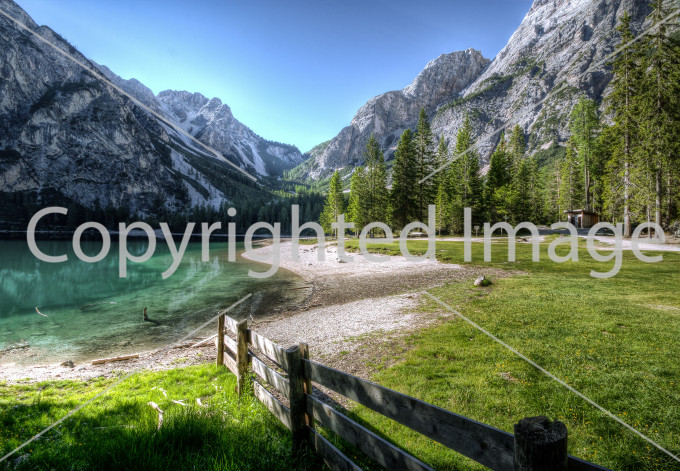\$img->insert(\$watermark, ‘center’);

``````// 插入圖片，從右下角起點，往左 10px，往上 50px
\$watermark = Image::make('public/watermark.png');
\$img->insert('public/watermark.png', 'bottom-right', 10, 50);
``````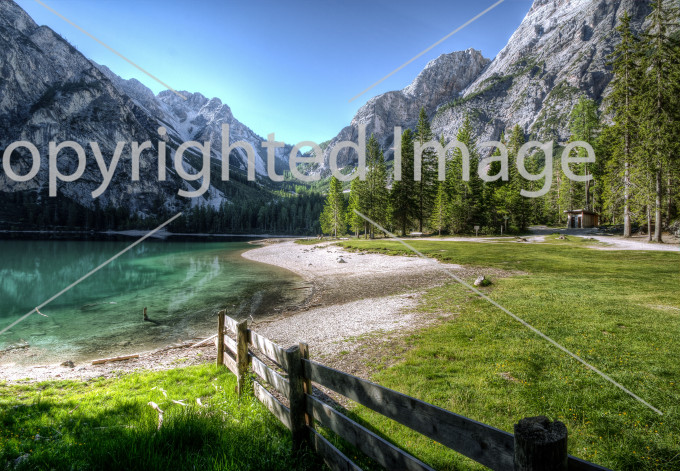\$img->insert(‘public/watermark.png’, ‘bottom-right’, 10, 50);

## invert() 反轉顏色

``````// 反轉圖片顏色
\$img = Image::make('public/foo.jpg')->invert();
``````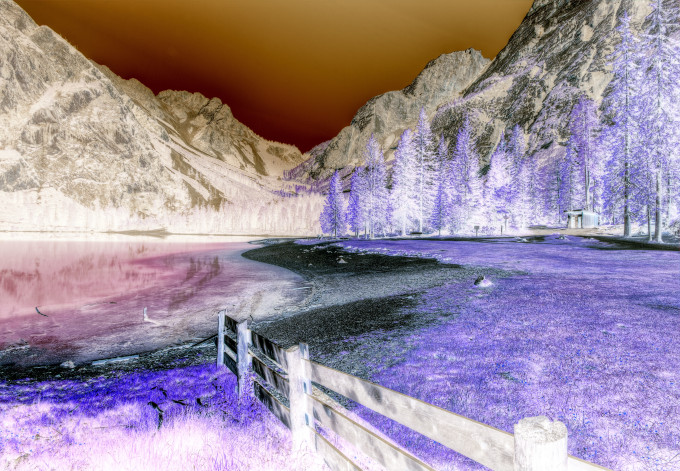\$img->invert();

## limitColors() 限制顯示顏色數量

``````// 建立圖片實例
\$img = Image::make('public/foo.png');
// 限制顏色數量為 100
\$img->limitColors(100);
``````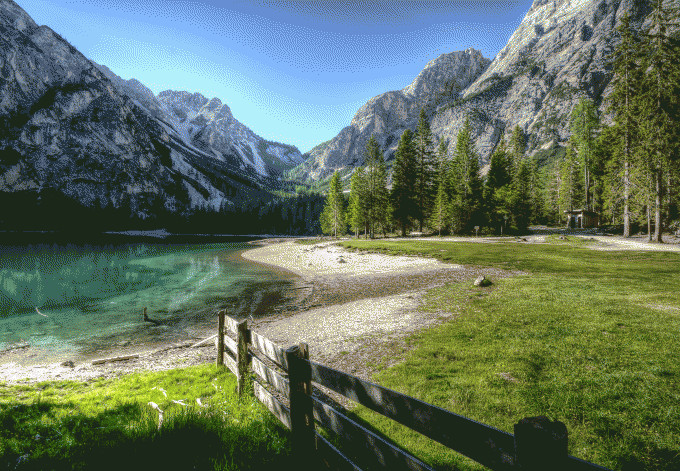\$img->limitColors(100);

``````// 限制顏色數量為 30
\$img->limitColors(30);
``````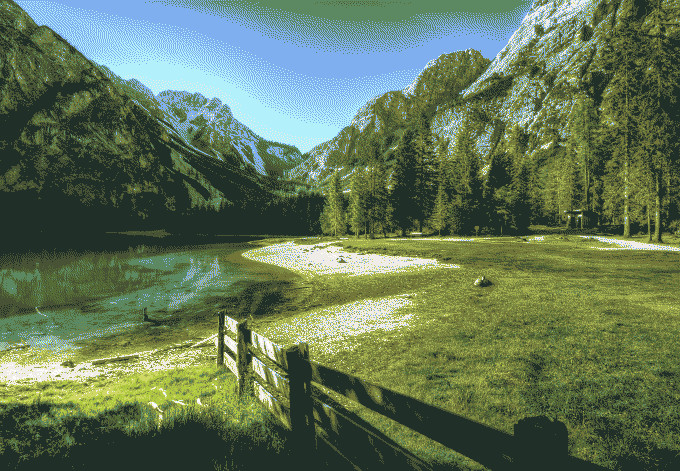\$img->limitColors(30);

## line() 畫線

``````// 建立圖片實例
\$img = Image::make('public/foo.png');

// 畫一條 5px 的紅線，從座標 (10,10) 到 (195, 195)
\$img->line(10, 10, 195, 195, function (\$draw) {
\$draw->color('#f00');
\$draw->width(5);
});
``````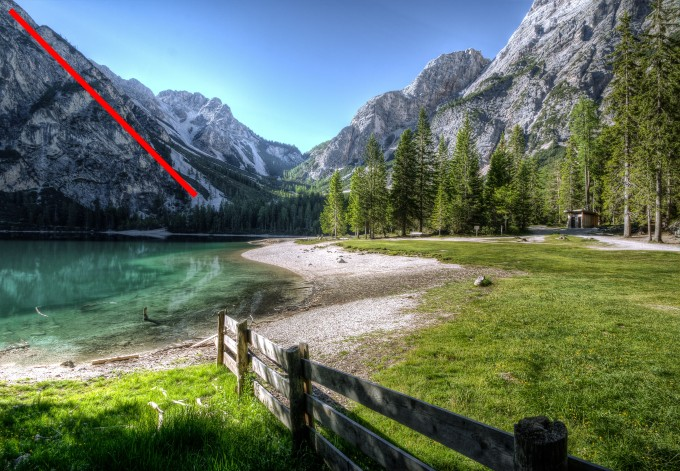\$img->line(10, 10, 195, 195) color #f00 width5

``````// 建立圖片實例
\$img = Image::make('public/foo.jpg');

// 建立圖片遮罩，有顏色的部分會為遮罩
``````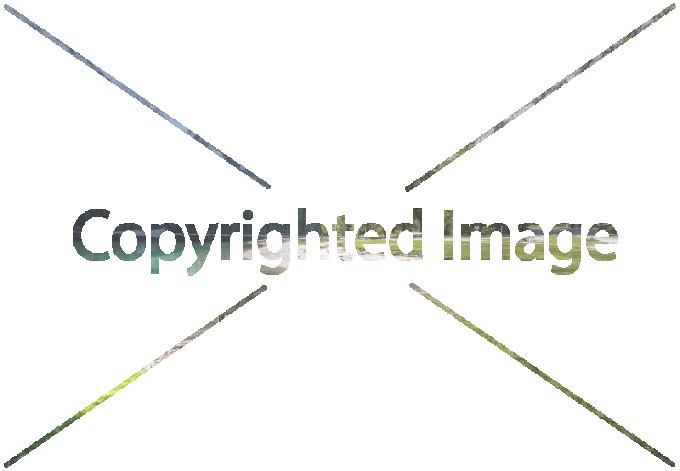``````// 建立圖片遮罩，有顏色且不為透明的部分會為遮罩
``````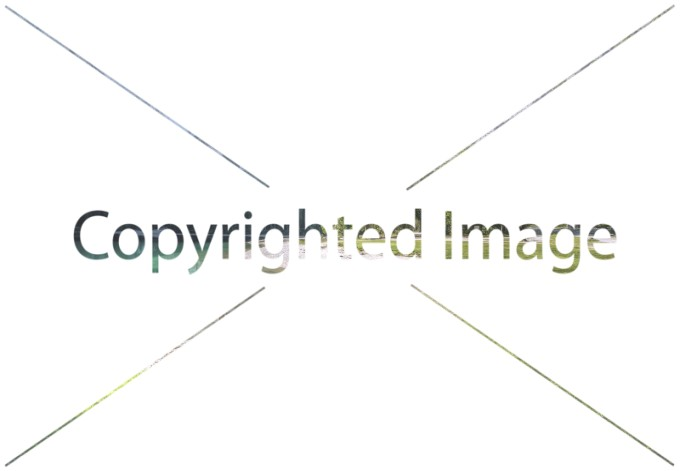## pixelate() 將圖片指定像素寬度像素化

``````// 建立圖片實例
\$img = Image::make('public/foo.jpg');

// 像素化效果:5px
\$img->pixelate(5);
``````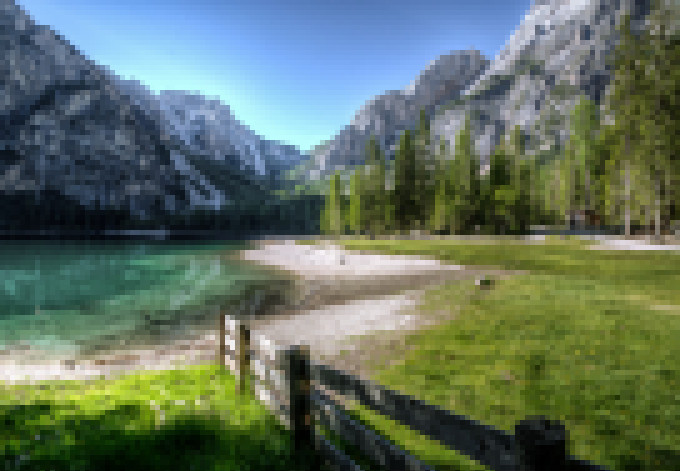\$img->pixelate(5);

``````// 像素化效果:12px
\$img->pixelate(12);
``````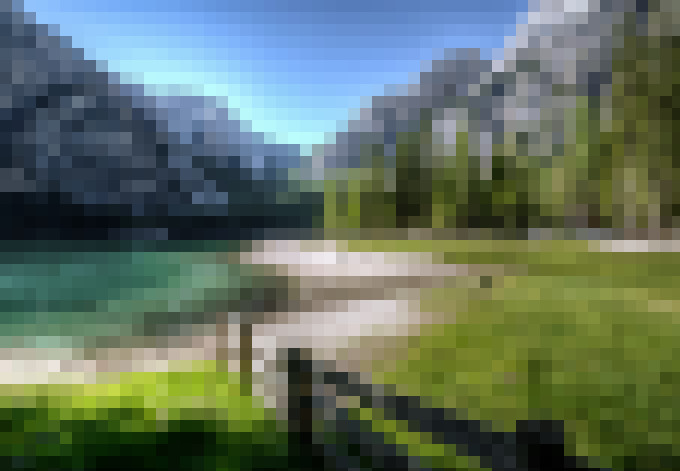\$img->pixelate(12);

``````// 像素化效果:30px
\$img->pixelate(30);
``````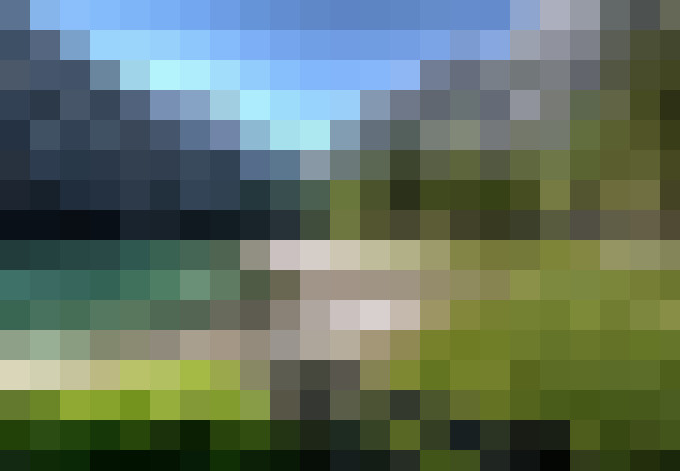\$img->pixelate(30);

## rotate() 旋轉圖片

``````// 建立圖片實例
\$img = Image::make('public/foo.jpg');

// 順時針旋轉圖圖片 45'
\$img->rotate(-45);
``````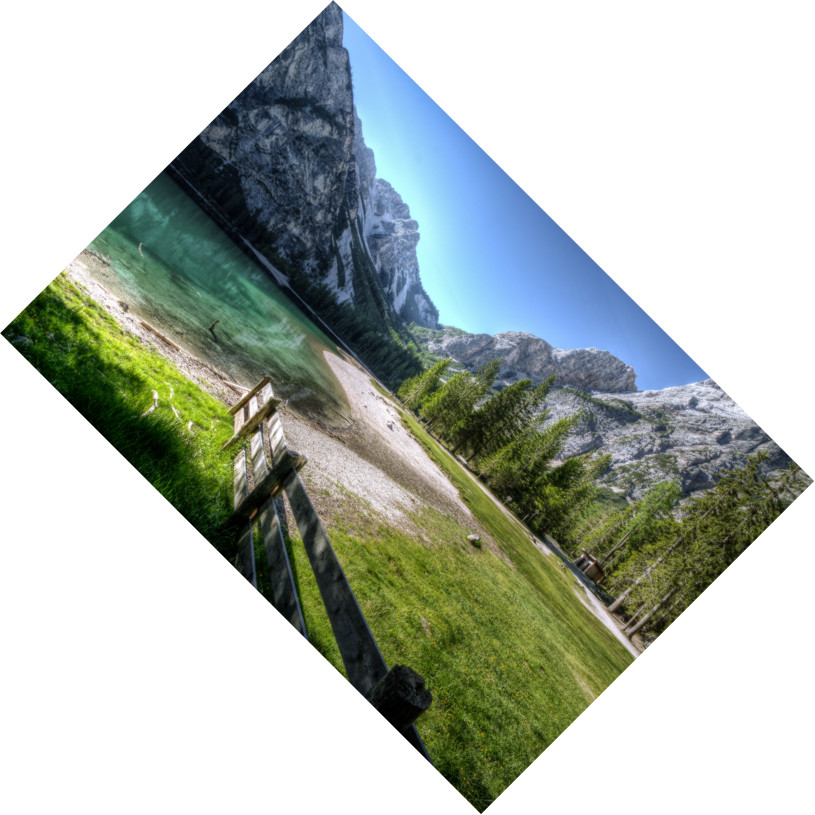\$img->rotate(-45);

## save() 儲存圖片

``````// 建立圖片實例
\$img = Image::make('public/foo.jpg');

// 儲存圖片，使用 60 品質的分數去儲存
\$img->save('public/bar.jpg', 60);

// 儲存相同的圖片
\$img->save('public/baz.jpg');

// 儲存 png 格式的圖片
\$img->save('public/bar.png');
``````

## sharpen() 銳利度

``````// 建立圖片實例
\$img = Image::make('public/foo.jpg');

// 銳利度 15
\$img->sharpen(15);
``````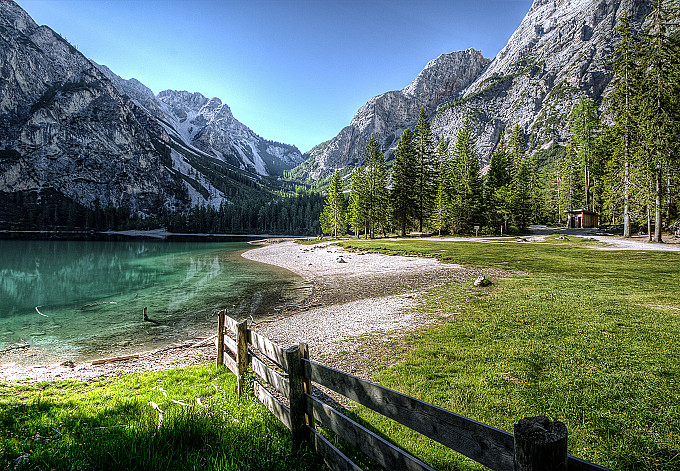\$img->sharpen(15);

``````// 銳利度 70
\$img->sharpen(70);
``````\$img->sharpen(70);

Laravel 是 PHP 的框架（Framework），提供了很多開發網站或 API 所需的工具及環境，經過簡單的設定就可以完成資料的處理及顯示，使開發者可以很優雅且快速的開發出各個不同的產品。本書適合有 PHP 基礎的人，但不知道要怎麼選擇框架，或者不用框架的人也能夠明白它的好處。### 購書連結